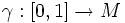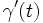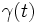# Geodesic for a linear connection

(diff) ← Older revision | Latest revision (diff) | Newer revision → (diff)
• A connected differential manifold$M$ with tangent bundle denoted by$TM$.
• A linear connection$\nabla$ for$M$.
Consider a smooth curve$\gamma:[0,1] \to M$. Consider the connection along$\gamma$ induced by$\nabla$, and consider the transport along$\gamma$ for that connection. Then, we say that$\gamma$ is a geodesic for$\nabla$ if, under that transport, the tangent vector$\gamma'(0)$ at$\gamma(0)$ gets transported, at time$t$, to the tangent vector$\gamma'(t)$ at$\gamma(t)$.